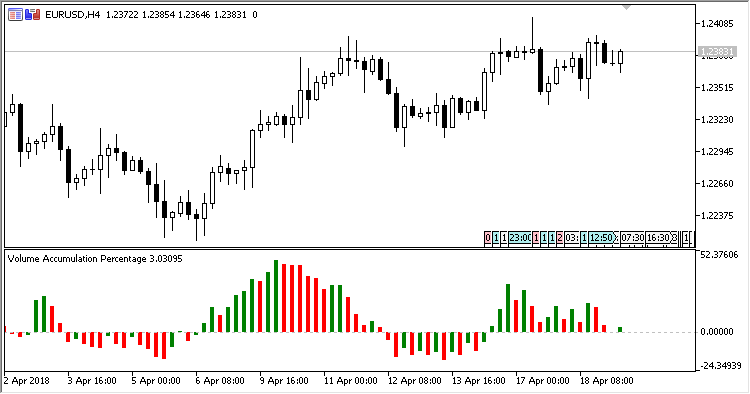# Volume_Accumulation_Percentage – indicator for MetaTrader 5

The Volume Accumulation oscillating indicator shows in a separate window the chart of candlestick volumes calculated by the following formula:

`VA = Volume * ((Close-Low)-(High-Close))/(High-Low)`

where:

```TVA= sum of VAs over a Period
TV = sum of Volume over a Period
VACC[Period] = (TVA / TV) * 100
```

The indicator has one input parameter:

• Period – calculation period.